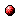# Max-plus algebra and max-plus linear discrete event systems: An introduction

Reference:
B. De Schutter and T. van den Boom, "Max-plus algebra and max-plus linear discrete event systems: An introduction," Proceedings of the 9th International Workshop on Discrete Event Systems (WODES'08), Göteborg, Sweden, pp. 36-42, May 2008.

Abstract:
We provide an introduction to the max-plus algebra and explain how it can be used to model a specific class of discrete event systems with synchronization but no concurrency. Such systems are called max-plus linear discrete event systems because they can be described by a model that is "linear" in the max-plus algebra. We discuss some key properties of the max-plus algebra and indicate how these properties can be used to analyze the behavior of max-plus linear discrete event systems. We also briefly present some control approaches for max-plus linear discrete event systems, including model predictive control. Finally, we discuss some extensions of the max-plus algebra and of max-plus linear systems.Corresponding technical report: pdf file (145 KB)
Note: More information on the pdf file format mentioned above can be found here.

Bibtex entry:

@inproceedings{DeSvan:08-007,
author={B. {D}e Schutter and T. van den Boom},
title={Max-plus algebra and max-plus linear discrete event systems: An introduction},
booktitle={Proceedings of the 9th International Workshop on Discrete Event Systems (WODES'08)},